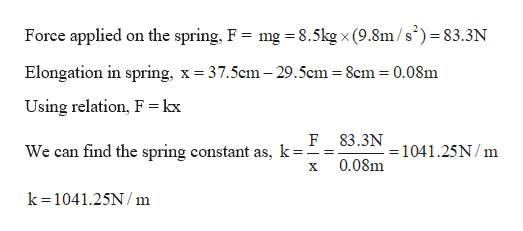# A 29.0 cm long spring is hung vertically from a ceiling and stretches to 37.5 cm when an 8.50 kg mass is hung from its free end. (a)Find the spring constant (in N/m). (b)Find the length of the spring (in cm) if the 8.50 kg weight is replaced with a 175 N weight.

Question
67 views
A 29.0 cm long spring is hung vertically from a ceiling and stretches to 37.5 cm when an 8.50 kg mass is hung from its free end.

(a)
Find the spring constant (in N/m).

(b)
Find the length of the spring (in cm) if the 8.50 kg weight is replaced with a 175 N weight.
check_circle

Step 1

Given:

Initial length of the spring = 29.5 cm

Weight from the spring = 8.5 kg weight

Final length of the spring = 37.5 cm

Step 2

Calculating the spring co...help_outlineImage TranscriptioncloseForce applied on the spring, F = mg = 8.5kg x (9.8m/s')=83.3N Elongation in spring, x = 37.5cm – 29.5cm = 8cm = 0.08m Using relation, F = kx F 83.3N We can find the spring constant as, k== =1041.25N/ m 0.08m k=1041.25N/m fullscreen

### Want to see the full answer?

See Solution

#### Want to see this answer and more?

Solutions are written by subject experts who are available 24/7. Questions are typically answered within 1 hour.*

See Solution
*Response times may vary by subject and question.
Tagged in
SciencePhysics

### Kinematics